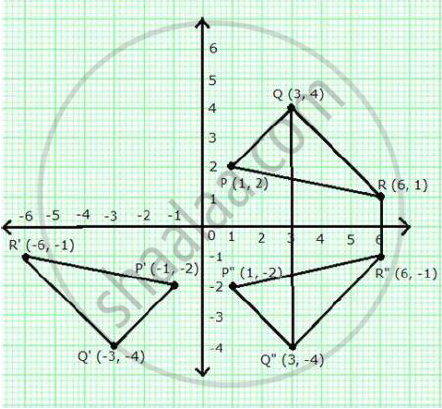# The points P (1, 2), Q (3, 4) and R (6, 1) are the vertices of PQR. (a) Write down the co-ordinates of P’, Q’ and R’, if P’Q’R’ is the image of PQR, when reflected in the origin. - Mathematics

The points P (1, 2), Q (3, 4) and R (6, 1) are the vertices of PQR.

(a) Write down the co-ordinates of P’, Q’ and R’, if P’Q’R’ is the image of PQR, when reflected in the origin.

(b) Write down the co-ordinates of P”, Q” and R”, if P”Q”R” is the image of PQR, when reflected in the x-axis.

(c) Mention the special name of the quadrilateral QRR”Q” and find its area.

#### Solution(a) The co-ordinates of P’, Q’ and R’ are (-1, -2), (-3, -4) and (-6, -1) respectively.

(b) The co-ordinates of P”, Q” and R” are (1, -2), (3, -4) and (6, -1) respectively.

(c) The quadrilateral QRR”Q” is an isosceles trapezium.

Area of QRR”Q” = ½ (RR”+ QQ”) × Height

= ½ (2 + 8) × 3 = 15 sq units

Concept: Invariant Points.
Is there an error in this question or solution?

#### APPEARS IN

Selina Concise Maths Class 10 ICSE
Chapter 12 Reflection
Exercise 12 (B) | Q 14 | Page 171